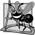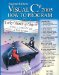``` 1 // Fig. 8.15: GradeBook.cs 2 // Grade book using an array to store test grades. 3 using System; 4 5 public class GradeBook 6 { 7 private string courseName; // name of course this GradeBook represents 8 private int[] grades; // array of student grades 9 10 // two-parameter constructor initializes courseName and grades array 11 public GradeBook( string name, int[] gradesArray ) 12 { 13 CourseName = name; // initialize courseName 14 grades = gradesArray; // initialize grades array 15 } // end two-parameter GradeBook constructor 16 17 // property that gets and sets the course name 18 public string CourseName 19 { 20 get 21 { 22 return courseName; 23 } // end get 24 set 25 { 26 courseName = value; 27 } // end set 28 } // end property CourseName 29 30 // display a welcome message to the GradeBook user 31 public void DisplayMessage() 32 { 33 // CourseName property gets the name of the course 34 Console.WriteLine( "Welcome to the grade book for {0}! ", 35 CourseName ); 36 } // end method DisplayMessage 37 38 // perform various operations on the data 39 public void ProcessGrades() 40 { 41 // output grades array 42 OutputGrades(); 43 44 // call method GetAverage to calculate the average grade 45 Console.WriteLine( " Class average is {0:F2}", GetAverage() ); 46 ``` 47 // call methods GetMinimum and GetMaximum 48 Console.WriteLine( "Lowest grade is {0} Highest grade is {1} ", 49 GetMinimum(), GetMaximum() ); 50 51 // call OutputBarChart to print grade distribution chart 52 OutputBarChart(); 53 } // end method ProcessGrades 54 55 // find minimum grade 56 public int GetMinimum() 57 { 58 int lowGrade = grades[ 0 ]; // assume grades[ 0 ] is smallest 59 60 // loop through grades array 61 foreach ( int grade in grades ) 62 { 63 // if grade lower than lowGrade, assign it to lowGrade 64 if ( grade < lowGrade ) 65 lowGrade = grade; // new lowest grade 66 } // end for 67 68 return lowGrade; // return lowest grade 69 } // end method GetMinimum 70 71 // find maximum grade 72 public int GetMaximum() 73 { 74 int highGrade = grades[ 0 ]; // assume grades[ 0 ] is largest 75 76 // loop through grades array 77 foreach ( int grade in grades ) 78 { 79 // if grade greater than highGrade, assign it to highGrade 80 if ( grade > highGrade ) 81 highGrade = grade; // new highest grade 82 } // end for 83 84 return highGrade; // return highest grade 85 } // end method GetMaximum 86 87 // determine average grade for test 88 public double GetAverage() 89 { 90 int total = 0; // initialize total 91 92 // sum grades for one student 93 foreach ( int grade in grades ) 94 total += grade; 95 96 // return average of grades 97 return ( double ) total / grades.Length; 98 } // end method GetAverage 99 100 // output bar chart displaying grade distribution 101 public void OutputBarChart() 102 { 103 Console.WriteLine( "Grade distribution:" ); 104 105 // stores frequency of grades in each range of 10 grades 106 int[] frequency = new int[ 11 ]; 107 108 // for each grade, increment the appropriate frequency 109 foreach ( int grade in grades ) 110 ++frequency[ grade / 10 ]; 111 112 // for each grade frequency, print bar in chart 113 for ( int count = 0; count < frequency.Length; count++ ) 114 { 115 // output bar label ( "00-09: ", ..., "90-99: ", "100: " ) 116 if ( count == 10 ) 117 Console.Write( " 100: " ); 118 else 119 Console.Write( "{0:D2}-{1:D2}: ", 120 count * 10, count * 10 + 9 ); 121 122 // print bar of asterisks 123 for ( int stars = 0; stars < frequency[ count ]; stars++ ) 124 Console.Write( "*" ); 125 126 Console.WriteLine(); // start a new line of output 127 } // end outer for 128 } // end method OutputBarChart 129 130 // output the contents of the grades array 131 public void OutputGrades() 132 { 133 Console.WriteLine( "The grades are: " ); 134 135 // output each student's grade 136 for ( int student = 0; student < grades.Length; student++ ) 137 Console.WriteLine( "Student {0,2}: {1,3}", 138 student + 1, grades[ student ] ); 139 } // end method OutputGrades 140 } // end class GradeBook

(This item is displayed on page 364 in the print version)

``` 1 // Fig. 8.16: GradeBookTest.cs
4 {
5 // Main method begins application execution
6 public static void Main( string[] args )
7 {
8 // one-dimensional array of student grades
9 int[] gradesArray = { 87, 68, 94, 100, 83, 78, 85, 91, 76, 87 };
10
12 "CS101 Introduction to C# Programming", gradesArray );
15 } // end Main
16 } // end class GradeBookTest
```

 ``` Welcome to the grade book for CS101 Introduction to C# Programming! The grades are: Student 1: 87 Student 2: 68 Student 3: 94 Student 4: 100 Student 5: 83 Student 6: 78 Student 7: 85 Student 8: 91 Student 9: 76 Student 10: 87 Class average is 84.90 Lowest grade is 68 Highest grade is 100 Grade distribution: 00-09: 10-19: 20-29: 30-39: 40-49: 50-59: 60-69: * 70-79: ** 80-89: **** 90-99: ** 100: * ```

Method ProcessGrades (lines 3953) contains a series of method calls that result in the output of a report summarizing the grades. Line 42 calls method OutputGrades to print the contents of array grades. Lines 136138 in method OutputGrades use a for statement to output the student grades. A for statement, rather than a foreach, must be used in this case, because lines 137138 use counter variable student's value to output each grade next to a particular student number (see Fig. 8.16). Although array indices start at 0, an instructor would typically number students starting at 1. Thus, lines 137138 output student + 1 as the student number to produce grade labels "Student 1: ", "Student2: " and so on.

Method ProcessGrades next calls method GetAverage (line 45) to obtain the average of the grades in the array. Method GetAverage (lines 8898) uses a foreach statement to total the values in array grades before calculating the average. The iteration variable in the foreach's header (e.g., int grade) indicates that for each iteration, int variable grade takes on a value in array grades. Note that the averaging calculation in line 97 uses grades.Length to determine the number of grades being averaged.

The application of Fig. 8.16 creates an object of class GradeBook (Fig. 8.15) using int array gradesArray (declared and initialized in line 9). Lines 1112 pass a course name and gradesArray to the GradeBook constructor. Line 13 displays a welcome message, and line 14 invokes the GradeBook object's ProcessGrades method. The output reveals the summary of the 10 grades in myGradeBook.## Software Engineering Observation 8 3

A test harness (or test application) is responsible for creating an object of the class being tested and providing it with data. This data could come from any of several sources. Test data can be placed directly into an array with an array initializer, it can come from the user at the keyboard, it can come from a file (as you will see in Chapter 18) or it can come from a network (as you will see in Chapter 23). After passing this data to the class's constructor to instantiate the object, the test harness should call the object to test its methods and manipulate its data. Gathering data in the test harness like this allows the class to manipulate data from several sources.

### Multidimensional ArraysVisual C# 2005 How to Program (2nd Edition)
ISBN: 0131525239
EAN: 2147483647
Year: 2004
Pages: 600

Similar book on Amazon